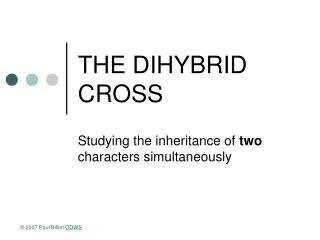DownloadDownload PresentationTHE DIHYBRID CROSS

# THE DIHYBRID CROSS

Télécharger la présentation## THE DIHYBRID CROSS

- - - - - - - - - - - - - - - - - - - - - - - - - - - E N D - - - - - - - - - - - - - - - - - - - - - - - - - - -
##### Presentation Transcript

1. THE DIHYBRID CROSS Studying the inheritance of two characters simultaneously © 2007 Paul Billiet ODWS

2. Mendel’s peas © 2007 Paul Billiet ODWS

3. Combinations © 2007 Paul Billiet ODWS

4. Is the inheritance of one character affected by the inheritance of another? © 2007 Paul Billiet ODWS

5. Is the inheritance of one character affected by the inheritance of another? © 2007 Paul Billiet ODWS

6. A dihybrid cross can be treated as two separate monohybrid crosses The expected probability of each type of seed can be calculated: • Probability of an F2 seed being round = 75% or ¾ • Probability of an F2 seed being wrinkled = • Probability of an F2 seed being yellow = • Probability of an F2 seed being green = © 2007 Paul Billiet ODWS

7. A dihybrid cross can be treated as two separate monohybrid crosses The expected probability of each type of seed can be calculated: • Probability of an F2 seed being round = 75% or ¾ • Probability of an F2 seed being wrinkled = 25% or ¼ • Probability of an F2 seed being yellow = 75% or ¾ • Probability of an F2 seed being green = 25% or ¼ © 2007 Paul Billiet ODWS

8. Therefore • Probability of an F2 seed being round and yellow = ¾ x ¾ = 9/16 = 56.25% • Probability of an F2 seed being round and green = • Probability of an F2 seed being wrinkled and yellow = • Probability of an F2 seed being wrinkled and green = © 2007 Paul Billiet ODWS

9. Therefore • Probability of an F2 seed being round and yellow = ¾ x ¾ = 9/16 = 56.25% • Probability of an F2 seed being round and green = ¾ x ¼ = 3/16 = 18.75% • Probability of an F2 seed being wrinkled and yellow = ¼ x ¾ = 3/16 = 18.75% • Probability of an F2 seed being wrinkled and green = ¼ x ¼ = 1/16= 6.25% © 2007 Paul Billiet ODWS

10. Predicting how many seeds we could expect to get in a sample © 2007 Paul Billiet ODWS

11. Predicting how many seeds we could expect to get in a sample © 2007 Paul Billiet ODWS

12. THE LAW OF INDEPENDENT ASSORTMENT • It appears that the inheritance of seed shape has no influence over the inheritance of seed colour • The two characters are inherited INDEPENDENTLY • The pairs of alleles that control these two characters assort themselves independently © 2007 Paul Billiet ODWS

13. Mendel & Meiosis • The pairs of chromosomes could orientate in different ways at Anaphase 1 © 2007 Paul Billiet ODWS

14. Dihybrid cross genetic diagram © 2007 Paul Billiet ODWS

15. Dihybrid cross genetic diagram © 2007 Paul Billiet ODWS

16. Dihybrid cross genetic diagram © 2007 Paul Billiet ODWS

17. Dihybrid cross proportions © 2007 Paul Billiet ODWS

18. Dihybrid cross proportions © 2007 Paul Billiet ODWS

19. Dihybrid test cross • In monohybrid crosses, to know if a dominant trait is homozygous (RR) or heterozygous (Rr) it is necessary to carry out a test cross • This is done with a homozygous recessive (rr) individual • The same is true for a dihybrid cross where the test cross is made with an individual which is homozygous recessive for both characters (rryy) © 2007 Paul Billiet ODWS

20. Dihybrid test cross © 2007 Paul Billiet ODWS